# AIIMS Chemistry Mock Test - 7

## 60 Questions MCQ Test AIIMS Mock Tests & Previous Year Papers | AIIMS Chemistry Mock Test - 7

Description
Attempt AIIMS Chemistry Mock Test - 7 | 60 questions in 60 minutes | Mock test for NEET preparation | Free important questions MCQ to study AIIMS Mock Tests & Previous Year Papers for NEET Exam | Download free PDF with solutions
QUESTION: 1

Solution:
QUESTION: 2

Solution:
QUESTION: 3

### Irrespective of the source, pure sample of water always yields 88.89% mass of oxygen and 11.11% mass of hydrogen. This is explained by the law of

Solution:
QUESTION: 4

The bond between two identical non metal atoms has a pair of electrons

Solution:
QUESTION: 5

Aromatic compounds undergo

Solution:
QUESTION: 6

The correct order of reactivity towards the electrophilic substitution of the compounds ,aniline (I),benzene (II) and nitrobenzene (III),is

Solution:
QUESTION: 7

Water gas is a mixture of

Solution:
QUESTION: 8

Gammexane is

Solution:
QUESTION: 9

Freon is used as a

Solution:
QUESTION: 10

The dimension of rate constant of a second order reaction involves

Solution:
QUESTION: 11

The entropy change when two moles of ideal monoatomic gas is heated from 200o C to 300o C reversibly and isochorically ?

Solution: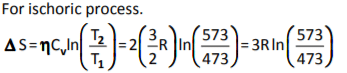QUESTION: 12

In the following question, a Statement of Assertion (A) is given followed by a corresponding Reason (R) just below it. Read the Statements carefully and mark the correct answer-
Assertion(A): The m.p./b.p. of noble gas are quite high.
Reason(R): The interparticle forces among noble gases in their liquid state are van der Waal's forces.

Solution:
QUESTION: 13

In the following question, a Statement of Assertion (A) is given followed by a corresponding Reason (R) just below it. Read the Statements carefully and mark the correct answer-
Assertion(A): Li⁺ ion has the lowest mobility in aqueous solutions among alkali metals.
Reason(R): Lithium has higher ionisation energy among alkali metals.

Solution:
QUESTION: 14

The reaction A → B follows first order kinetics. The time taken for 0.8 mole of A to produce 0.6 mole of B is 1 hour. What is the time taken for conversion of 0.9 mole of A to produce 0.675 moles of B?

Solution:
QUESTION: 15

In the following question, a Statement of Assertion (A) is given followed by a corresponding Reason (R) just below it. Read the Statements carefully and mark the correct answer-
Assertion(A): The reaction rate of most of the reactions doubles when temperature is increased from 298 K to 308 K.
Reason(R): The activation energy of reaction decreases with increase in temperature.

Solution:
QUESTION: 16

In the following question, a Statement of Assertion (A) is given followed by a corresponding Reason (R) just below it. Read the Statements carefully and mark the correct answer-
Assertion(A): The oxidation state of iron in brown coloured complex formed in ring test is +1.
Reason(R): The measured magnetic moment is 3.87 BM.

Solution:
QUESTION: 17

In the following question, a Statement of Assertion (A) is given followed by a corresponding Reason (R) just below it. Read the Statements carefully and mark the correct answer-
Assertion(A): Second ionisation energy of C,N,O,F, increases in the order. C < N < F < O.
Reason (R): Ionisation energy increase with deceasing size.

Solution:
QUESTION: 18

In the following question, a Statement of Assertion (A) is given followed by a corresponding Reason (R) just below it. Read the Statements carefully and mark the correct answer-
Assertion(A): Iodination of alkane is made in presence of HgO or HIO3.
Reason(R): Iodination is very slow and reversible process.

Solution:

The reaction of I2 with alkanes is reversible because the HI formed as a by-product is a moderate reducing agent and hence reduces the iodoalkane back to alkane.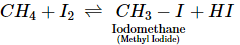Thus, the iodination can be carried out in presence of an oxidising agent such as iodic acid (HIO3) or HgO which converts HI to I2 as it is formed: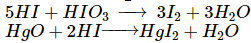QUESTION: 19

In the following question, a Statement of Assertion (A) is given followed by a corresponding Reason (R) just below it. Read the Statements carefully and mark the correct answer-
Assertion(A): [Ni(CN)4]2- has square planar and [NiCl4]2- has tetrahedral shape.
Reason(R): [Ni(CN)4]2- is diamagnetic while NiCl22- is paramagnetic.

Solution:
QUESTION: 20

In the following question, a Statement of Assertion (A) is given followed by a corresponding Reason (R) just below it. Read the Statements carefully and mark the correct answer-
Assertion(A): Equanil is a tranquiliser.
Reason(R): Equanil is used to cure depression and hypertension.

Solution:
QUESTION: 21

In the following question, a Statement of Assertion (A) is given followed by a corresponding Reason (R) just below it. Read the Statements carefully and mark the correct answer-
Assertion(A): Boric acid acts as a strong acid in presence of catechol.
Reason(R): Boric acid does not act as a proton donor but behaves as a Lewis acid.

Solution:
QUESTION: 22

In the following question, a Statement of Assertion (A) is given followed by a corresponding Reason (R) just below it. Read the Statements carefully and mark the correct answer-
Assertion(A): Ice is less dense than water.
Reason(R): Ice is a solid whereas water is liquid.

Solution:
QUESTION: 23

In the following question, a Statement of Assertion (A) is given followed by a corresponding Reason (R) just below it. Read the Statements carefully and mark the correct answer-
Assertion(A): Electrons enter into fifth shell even before the fourth shell is completely filled.
Reason(R): The subshell with lower value of (n + 1) possesses higher energy.

Solution:
QUESTION: 24

In the following question, a Statement of Assertion (A) is given followed by a corresponding Reason (R) just below it. Read the Statements carefully and mark the correct answer-
Assertion(A): According to the principle of common ion effect, the solubility of HgI₂ is expected to be less in an aqueous solution of KI than in water. But HgI₂ dissolves in an aqueous solution of KI to form a clear solution.
Reason(R): Iodide ion, I⁻, is highly polarisable.

Solution:
QUESTION: 25

In the following question, a Statement of Assertion (A) is given followed by a corresponding Reason (R) just below it. Read the Statements carefully and mark the correct answer-
Assertion(A): The probability of finding 4s-electron very close to nucleus is not zero.
Reason(R): 4s electron density can penetrate into the inner shell electron density.

Solution:
QUESTION: 26

In the following question, a Statement of Assertion (A) is given followed by a corresponding Reason (R) just below it. Read the Statements carefully and mark the correct answer-
Assertion(A): Non-metals oxidation state of metal
Reason(R): Oxidation state of metal becomes positive as it is oxidised and non-metal becomes negative asitis reduced.

Solution:
QUESTION: 27

In the following question, a Statement of Assertion (A) is given followed by a corresponding Reason (R) just below it. Read the Statements carefully and mark the correct answer-
Assertion(A): Elastomers are polymers that will not stretch when a force is applied on them.
Reason(R): Rubber is an elastomer.

Solution:
QUESTION: 28

In the following question, a Statement of Assertion (A) is given followed by a corresponding Reason (R) just below it. Read the Statements carefully and mark the correct answer-
Assertion(A): Electronegativity of alkalinmi metal is low.
Reason(R): Ionisation energy and electron gain ehthalpy of alkali metal is low.

Solution:
QUESTION: 29

In the following question, a Statement of Assertion (A) is given followed by a corresponding Reason (R) just below it. Read the Statements carefully and mark the correct answer-
Assertion(A): In CaCl crystal, the co-ordination number of Cs+ ion is 8.
Reason(R): Ci-ion in CsCl adopt bcc type of packing.

Solution:
QUESTION: 30

In the following question, a Statement of Assertion (A) is given followed by a corresponding Reason (R) just below it. Read the Statements carefully and mark the correct answer-
Assertion(A): The rest mass for electron is 0.0005486 u.
Reason (R): Electron is assumed moving while calculating rest mass.

Solution:
QUESTION: 31

In the following question, a Statement of Assertion (A) is given followed by a corresponding Reason (R) just below it. Read the Statements carefully and mark the correct answer-
Assertion(A): CH₃ is an antiseptic agent.
Reason(R): CH₃ is volatilisable.

Solution:
QUESTION: 32

In the following question, a Statement of Assertion (A) is given followed by a corresponding Reason (R) just below it. Read the Statements carefully and mark the correct answer-
Assertion(A): [Pt(NH2)2Cl2] is tetrahedral.
Reason(R): The oxidation state of platinum is +2.

Solution:
QUESTION: 33

The standard reduction potentials of Ag, Cu, Co and Zn are 0.799, 0.337, -0.277 and -0.762 V respectively. Which of the following cells will have maximum cell EMF?

Solution:
QUESTION: 34

Calculate the amount of charge flowing in 2 minute in a wire of resistance 10 Ω when a potential difference of 20 V is applied

Solution:
QUESTION: 35

Gases show ideal behaviour at

Solution:
QUESTION: 36

The alkali metal that reacts with nitrogen directly to form nitride is

Solution:
QUESTION: 37

Main process for the manufacture of Na₃CO₃ is

Solution:
QUESTION: 38

Which of the following imparts violet colouration to the Bunsen burner nonluminous flame?

Solution:
QUESTION: 39

The halogen which is most easily reduced is

Solution:
QUESTION: 40

The effective compound of bleaching powder is

Solution:
QUESTION: 41

Heavy water is used as

Solution:
QUESTION: 42

Which has the highest pH ?

Solution:
QUESTION: 43

Hydrogen ion concentration of 0.2 M CH₃COOH, which is 40% dissociated, is

Solution:
QUESTION: 44

Which of the following sulphides has the lowest solubility product?

Solution:
QUESTION: 45

The conjugate base of H₂PO₄⁻ is

Solution:
QUESTION: 46

The IUPAC name of acetone is

Solution:
QUESTION: 47

Reduction with aluminium isopropoxide in excess of Isopropyl alcohol is called Meerwein Ponndroff-Verley reduction. What will be the final product when cyclohex-2-enone is selectively reduced in MPV reaction ?

Solution:
QUESTION: 48

Which group decreases acidic nature of phenol?

Solution:
QUESTION: 49

In the following conjugated system
CH3CH═CHCH═CHCH3 addition is considered at the :

Solution:
QUESTION: 50

Select incorect statement(s):

Solution:
QUESTION: 51

Indicate the alkyl halides out of the following which can undergo SN1 reaction :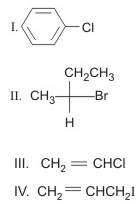Solution:
QUESTION: 52

Carborundum is formed by the reaction of

Solution:
QUESTION: 53

Which of the following glass is used in making wind screen of automobiles?

Solution:
QUESTION: 54

The edge length of the unit cell of body-centred lattice is 500 pm. If the radius of cation is 169 pm, then what is radius of anion?

Solution:
QUESTION: 55

For a dilute solution, Raoult's law states that

Solution:
QUESTION: 56

Identify the mixture that shows positive deviations from Raoult's law

Solution:
QUESTION: 57

5 ml of N HCl, 20 ml of N/2 H₂SO₄ and 30 ml N/3 HNO₃ are mixed together, the volume made to one litre. The normality of the resulting solution is

Solution:
QUESTION: 58

In a balanced equation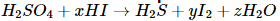the values of x,y and z are

Solution:
QUESTION: 59

How many 'd' electrons are present in Cr2⁺ ion ?

Solution:
QUESTION: 60

Which product (s) would form in the following reaction?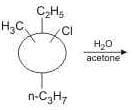Solution:Use Code STAYHOME200 and get INR 200 additional OFF Use Coupon Code

### How to Prepare for NEET

Read our guide to prepare for NEET which is created by Toppers & the best Teachers